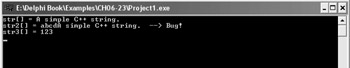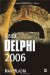C Strings

C++ Strings

The C++ language, unlike Delphi, doesn't have a native string type. The closest thing the C++ language has to a string is an array of characters:

char array_name[number_of_chars];

Even though the C++ language doesn't have a proper string data type, C++Builder has the AnsiString data type, which is equivalent to the Delphi string data type.

In this chapter, and other chapters that deal with the C++ language, we're going to use character arrays. Starting with Chapter 11, which is the first chapter that covers VCL Forms programming for the Win32 platform, we're mainly going to use the string data type in Delphi (as always) and the AnsiString type in C++Builder applications.

Initializing Strings

In C++, you can initialize a character array as you would other arrays, or you can initialize a character array by passing a string literal to it. Here are three different initializations:

char str[] = "A simple C++ string."; char str2[] = {'a', 'b', 'c', 'd'}; char str3[] = {'1', '2', '3', '\0'}; char str4;

The first way of initializing character arrays is the best way since it involves the least typing and because the compiler implicitly adds the null character at the end of the string. The null character (\0) is absolutely necessary since strings in C++, like the string data type in Delphi, are null-terminated.

The second array is initialized improperly. Although the code compiles perfectly, it will produce errors at run time (see Figure 6-4) since there's no null character at the end of the array.Figure 6-4: Using character arrays

The str3 array shows how to properly initialize a character array if you really want to initialize it char by char, and the last array illustrates how to explicitly define the length of the character array. Note that the str4 array can only accept four characters. The last element must be reserved for the null character.

The following listing contains code that displays the above character arrays on screen. Notice what happens when you use a character array without a null character at its end.

Listing 6-23: Using character arrays#include <iostream.h> #include <conio.h> #include <string.h> #pragma hdrstop #pragma argsused int main(int argc, char* argv[]) {    char str[] = "A simple C++ string.";    char str2[] = {'a', 'b', 'c', 'd'};    char str3[] = {'1', '2', '3', '\0'};    char str4;    cout << "str[] = " << str <<  endl       << "str2[] = " << str2 << "   --> Bug!" << endl       << "str3[] = " << str3 << endl;    getch();    return 0; }Assigning Values to Strings

To assign a value to a character array, you cannot use the assignment operator. Instead, you have to use the strcpy function declared in the string.h header file. The strcpy function accepts two character array parameters. The first one is the destination array and the second is, obviously, the source character array.

Here's how to assign a value to a character array (string) in C++:

char name;  strcpy(name, "Santa Claus");

If you want to, you can also assign a value to a character array like this:

name = 'S'; name = 'a'; name = 'n'; name = 't'; name = 'a'; name = '\0'; cout << name << endl;

String Related Functions

Commonly used string manipulation functions are declared in two header files: string.h and stdlib.h. The string.h header file contains manipulation functions, and the stlib.h header file contains functions for converting strings to integers and back.

Table 6-1: Common string manipulation functions

C++ Function

Delphi
Equivalent

Description

strcpy(dest, src)

=, Copy

string.h

Copies src to dest

strcat(dest, src)

+, Concat

string.h

Adds src to the end of dest

strlen(string)

Length

string.h

Gets length of string

strcmp(str1, str2)

=, <>

string.h

Compares two strings

strchr(string, char)

Pos

string.h

Finds char in string

strstr(string, substring)

Pos

string.h

Finds substring in string

int atoi(string)

StrToInt

stdlib.h

Converts string to integer

itoa(int, char[], int)

IntToStr

stdlib.h

Converts integer to string

Listing 6-24 illustrates how to use the common functions listed in Table 6-1.

Listing 6-24: Using common string functions#include <iostream.h> #include <conio.h> #include <string.h> #include <stdlib.h> #pragma hdrstop #pragma argsused int main(int argc, char* argv[]) {    char test = "ab";    char name;    strcpy(name, "Santa Claus");    /* comparing and copying */    if(strcmp(name, test) == 0)       cout << "Strings are the same." << endl;    else {       cout << "Strings are different." << endl;       strcpy(test, name);       if(strcmp(name, test) == 0)         cout << "Now they're the same." << endl;    }    /* finding a substring */    if(strstr(name, "Santa") != 0) {       cout << "I found Santa!" << endl;    }    /* get length, concatenate, */    char empty = {'\0'};    if(strlen(empty) == 0) {       strcat(empty, "abc");       strcat(empty, "def");       cout << empty << endl;    }    /* convert string to int */    char s[] = "101";    int x = atoi(s);    cout << "x = " << x <<  endl;    /* convert int to string, itoa(int, dest_array, radix) */    int y = 2005;    char dest;    itoa(y, dest, 10); // 10 for decimal    cout << "y = " <<  dest << endl;    getch();    return 0; }Inside Delphi 2006 (Wordware Delphi Developers Library)
ISBN: 1598220039
EAN: 2147483647
Year: 2004
Pages: 212